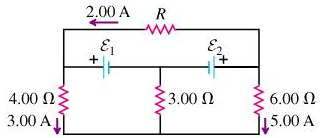# Problem: Find the resistance R. (Note that three currents are given.)

###### FREE Expert Solution

We'll use Kirchoff's loop rule.

Using the top loop:

-2.00R - ε1 + ε2 = 0

95% (333 ratings)###### Problem DetailsFind the resistance R. (Note that three currents are given.)